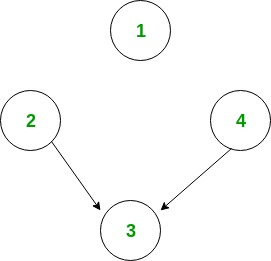# Number of sink nodes in a graph

• Difficulty Level : Basic
• Last Updated : 29 Nov, 2021

Given a Directed Acyclic Graph of n nodes (numbered from 1 to n) and m edges. The task is to find the number of sink nodes. A sink node is a node such that no edge emerges out of it.

Examples:

Attention reader! Don’t stop learning now. Get hold of all the important DSA concepts with the DSA Self Paced Course at a student-friendly price and become industry ready.  To complete your preparation from learning a language to DS Algo and many more,  please refer Complete Interview Preparation Course.

In case you wish to attend live classes with experts, please refer DSA Live Classes for Working Professionals and Competitive Programming Live for Students.

```Input : n = 4, m = 2
Edges[] = {{2, 3}, {4, 3}}
Output : 2``````Only node 1 and node 3 are sink nodes.

Input : n = 4, m = 2
Edges[] = {{3, 2}, {3, 4}}
Output : 3```

The idea is to iterate through all the edges. And for each edge, mark the source node from which the edge emerged out. Now, for each node check if it is marked or not. And count the unmarked nodes.

Algorithm:

```
1. Make any array A[] of size equal to the
number of nodes and initialize to 1.
2. Traverse all the edges one by one, say,
u -> v.
(i) Mark A[u] as 1.
3. Now traverse whole array A[] and count
number of unmarked nodes.```

Below is implementation of this approach:

## C++

 `// C++ program to count number if sink nodes``#include``using` `namespace` `std;`` ` `// Return the number of Sink NOdes.``int` `countSink(``int` `n, ``int` `m, ``int` `edgeFrom[],``                        ``int` `edgeTo[])``{``    ``// Array for marking the non-sink node.``    ``int` `mark[n];``    ``memset``(mark, 0, ``sizeof` `mark);`` ` `    ``// Marking the non-sink node.``    ``for` `(``int` `i = 0; i < m; i++)``        ``mark[edgeFrom[i]] = 1;`` ` `    ``// Counting the sink nodes.``    ``int` `count = 0;``    ``for` `(``int` `i = 1; i <= n ; i++)``        ``if` `(!mark[i])``            ``count++;`` ` `    ``return` `count;``}`` ` `// Driven Program``int` `main()``{``    ``int` `n = 4, m = 2;``    ``int` `edgeFrom[] = { 2, 4 };``    ``int` `edgeTo[] = { 3, 3 };`` ` `    ``cout << countSink(n, m, edgeFrom, edgeTo) << endl;`` ` `    ``return` `0;``}`

## Java

 `// Java program to count number if sink nodes``import` `java.util.*;`` ` `class` `GFG``{`` ` `// Return the number of Sink NOdes.``static` `int` `countSink(``int` `n, ``int` `m, ``                     ``int` `edgeFrom[], ``int` `edgeTo[])``{``    ``// Array for marking the non-sink node.``    ``int` `[]mark = ``new` `int``[n + ``1``];`` ` `    ``// Marking the non-sink node.``    ``for` `(``int` `i = ``0``; i < m; i++)``        ``mark[edgeFrom[i]] = ``1``;`` ` `    ``// Counting the sink nodes.``    ``int` `count = ``0``;``    ``for` `(``int` `i = ``1``; i <= n ; i++)``        ``if` `(mark[i] == ``0``)``            ``count++;`` ` `    ``return` `count;``}`` ` `// Driver Code``public` `static` `void` `main(String[] args)``{``    ``int` `n = ``4``, m = ``2``;``    ``int` `edgeFrom[] = { ``2``, ``4` `};``    ``int` `edgeTo[] = { ``3``, ``3` `};`` ` `    ``System.out.println(countSink(n, m, ``                       ``edgeFrom, edgeTo));``}``}`` ` `// This code is contributed by 29AjayKumar`

## Python3

 `# Python3 program to count number if sink nodes`` ` `# Return the number of Sink NOdes. ``def` `countSink(n, m, edgeFrom, edgeTo):``     ` `    ``# Array for marking the non-sink node. ``    ``mark ``=` `[``0``] ``*` `(n ``+` `1``)`` ` `    ``# Marking the non-sink node.``    ``for` `i ``in` `range``(m):``        ``mark[edgeFrom[i]] ``=` `1`` ` `    ``# Counting the sink nodes. ``    ``count ``=` `0``    ``for` `i ``in` `range``(``1``, n ``+` `1``):``        ``if` `(``not` `mark[i]): ``            ``count ``+``=` `1`` ` `    ``return` `count`` ` `# Driver Code``if` `__name__ ``=``=` `'__main__'``: ``     ` `    ``n ``=` `4``    ``m ``=` `2``    ``edgeFrom ``=` `[``2``, ``4``] ``    ``edgeTo ``=` `[``3``, ``3``]`` ` `    ``print``(countSink(n, m, edgeFrom, edgeTo))`` ` `# This code is contributed by PranchalK`

## C#

 `// C# program to count number if sink nodes``using` `System;``     ` `class` `GFG``{`` ` `// Return the number of Sink NOdes.``static` `int` `countSink(``int` `n, ``int` `m, ``                     ``int` `[]edgeFrom,``                     ``int` `[]edgeTo)``{``    ``// Array for marking the non-sink node.``    ``int` `[]mark = ``new` `int``[n + 1];`` ` `    ``// Marking the non-sink node.``    ``for` `(``int` `i = 0; i < m; i++)``        ``mark[edgeFrom[i]] = 1;`` ` `    ``// Counting the sink nodes.``    ``int` `count = 0;``    ``for` `(``int` `i = 1; i <= n ; i++)``        ``if` `(mark[i] == 0)``            ``count++;`` ` `    ``return` `count;``}`` ` `// Driver Code``public` `static` `void` `Main(String[] args)``{``    ``int` `n = 4, m = 2;``    ``int` `[]edgeFrom = { 2, 4 };``    ``int` `[]edgeTo = { 3, 3 };`` ` `    ``Console.WriteLine(countSink(n, m, ``                      ``edgeFrom, edgeTo));``}``}`` ` `// This code is contributed by PrinciRaj1992`

## Javascript

 ``

Output:

`2`

Time Complexity: O(m + n) where n is number of nodes and m is number of edges.

Related Article:
The Celebrity Problem

This article is contributed by Anuj Chauhan. If you like GeeksforGeeks and would like to contribute, you can also write an article using write.geeksforgeeks.org or mail your article to review-team@geeksforgeeks.org. See your article appearing on the GeeksforGeeks main page and help other Geeks.